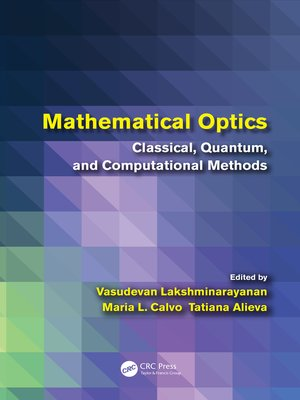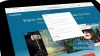Mathematical Optics

Classical, Quantum, and Computational Methods

byVasudevan LakshminarayananEditor · María L. CalvoEditor

ebook

With an OverDrive account, you can save your favorite libraries for at-a-glance information about availability. Find out more about OverDrive accounts.

Subjects

Going beyond standard introductory texts, Mathematical Optics: Classical, Quantum, and Computational Methods brings together many new mathematical techniques from optical science and engineering research. Profusely illustrated, the book makes the material accessible to students and newcomers to the field.

Divided into six parts, the text presents state-of-the-art mathematical methods and applications in classical optics, quantum optics, and image processing.

• Part I describes the use of phase space concepts to characterize optical beams and the application of dynamic programming in optical waveguides.
• Part II explores solutions to paraxial, linear, and nonlinear wave equations.
• Part III discusses cutting-edge areas in transformation optics (such as invisibility cloaks) and computational plasmonics.
• Part IV uses Lorentz groups, dihedral group symmetry, Lie algebras, and Liouville space to analyze problems in polarization, ray optics, visual optics, and quantum optics.
• Part V examines the role of coherence functions in modern laser physics and explains how to apply quantum memory channel models in quantum computers.
• Part VI introduces super-resolution imaging and differential geometric methods in image processing.
• As numerical/symbolic computation is an important tool for solving numerous real-life problems in optical science, many chapters include Mathematica® code in their appendices. The software codes and notebooks as well as color versions of the book's figures are available at www.crcpress.com.

Publication Details

Publisher:
CRC Press
Publication Date:
2018

Format